﻿ Common Core Standards for Math - Kindergarten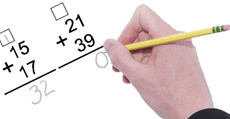Adaptive Worksheets

Helping Every Child to Succeed

toll-free (888) 777-0876
fax (888) 777-0875

## Generalize place value understanding for multi-digit whole numbers

4.NBT.A.1
Recognize that in a multi-digit whole number, a digit in one place represents ten times what it represents in the place to its right.Place Value in Multi-Digit Numbers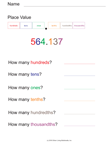Place Value Including Tenths and Hundredths

4.NBT.A.2

Read and write multi-digit whole numbers using base-ten numerals, number names, and expanded form. Compare two multi-digit numbers based on meanings of the digits in each place, using >, =, and < symbols to record the results of comparisons.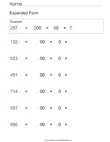Expanded FormExpanded Form with Visual Prompts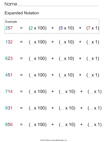Expanded Notation

4.NBT.A.3
Use place value understanding to round multi-digit whole numbers to any place.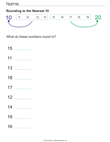Rounding To the Nearest 10Rounding With Prompts to the Nearest 100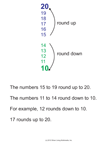Rounding to the Nearest 10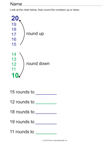Rounding to the Nearest 10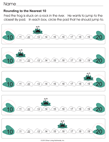Rounding with Frog PromptsRounding with Frog Prompts

## 4.NBT.B.4 Fluently add and subtract multi-digit whole numbers using the standard algorithm.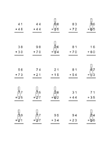Multidigit Addition Worksheet Generator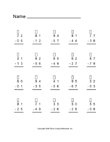Multidigit Subtraction Worksheet Generator

## 4.NBT.B.5

Multiply a whole number of up to four digits by a one-digit whole number, and multiply two 2-digit numbers, using strategies based on place value and the properties of operations. Illustrate and explain the calculation by using equations, rectangular arrays, and/or area models.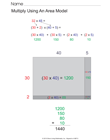Use an Area Model to MultiplyMultiply Rectangular ArraysSimple Multiplication Arrays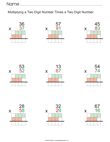Multiply Two 2-Digit Numbers with Visual PromptsMultiply a 3-Digit Number by a 2-Digit Number  with Visual PromptsMultiplication by 2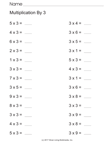Multiplication by 3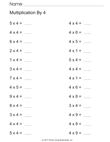Multiplication by 4Multiplication by 5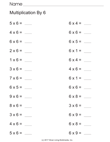Multiplication by 6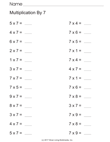Multiplication by 7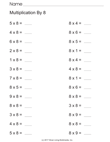Multiplication by 8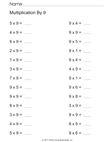Multiplication by 9Vertical Multiplication By 1Vertical Multiplication By 2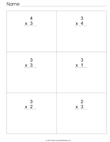Vertical Multiplication By 3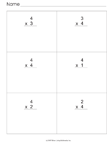Vertical Multiplication By 4Vertical Multiplication By 5Vertical Multiplication By 6Vertical Multiplication By 7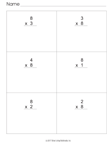Vertical Multiplication By 8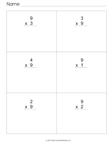Vertical Multiplication By 9Vertical Multiplication By 10Vertical Multiplication By 11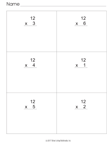Vertical Multiplication By 12

4.NBT.B.6
Find whole-number quotients and remainders with up to four-digit dividends and one-digit divisors, using strategies based on place value, the properties of operations, and/or the relationship between multiplication and division. Illustrate and explain the calculation by using equations, rectangular arrays, and/or area models.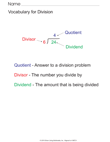Division VocabularyLong Division Explanation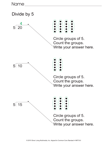Long Division By 5 With VisualsLong Division By 5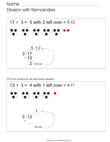Long Division By 3 With Remainders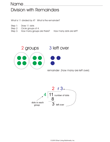Long Division with Remainders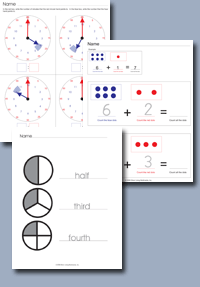Click on any of the standards on the left to create custom worksheets or to print premade materials.